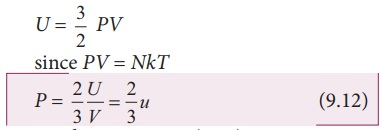Home | | Physics 11th std | Relation between pressure and mean kinetic energy

# Relation between pressure and mean kinetic energy

Physics : Kinetic Theory of Gases - Relation between pressure and mean kinetic energy

Relation between pressure and mean kinetic energy

From earlier section, the internal energy of the gas is given byThe above equation can also be written asFrom the equation (9.12), we can state that the pressure of the gas is equal to two thirds of internal energy per unit volume or internal energy density (u=U/V).

Writing pressure in terms of mean kinetic energy density using equation (9.6)where ρ = nm = mass density (Note n is number density)

Multiply and divide R.H.S of equation (9.13) by 2, we getFrom the equation (9.14), pressure is equal to 2/3 of mean kinetic energy per unit volume.

Study Material, Lecturing Notes, Assignment, Reference, Wiki description explanation, brief detail
11th Physics : UNIT 9 : Kinetic Theory of Gases : Relation between pressure and mean kinetic energy |